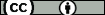## On the Gaussian behavior of marginals and the mean width of random polytopes

Alonso Gutiérrez, David (Universidad de Zaragoza) ; Prochno, Joscha
Resumen: We show that the expected value of the mean width of a random polytope generated by $N$ random vectors ( $n\leq N\leq e^{\sqrt n}$) uniformly distributed in an isotropic convex body in $\mathbb{R}^n$ is of the order $\sqrt {\log N} L_K$. This completes a result of Dafnis, Giannopoulos and Tsolomitis. We also prove some results in connection with the 1-dimensional marginals of the uniform probability measure on an isotropic convex body, extending the interval in which the average of the distribution functions of those marginals behaves in a sub- or supergaussian way.
Idioma: Inglés
DOI: 10.1090/S0002-9939-2014-12401-4
Año: 2015
Publicado en: PROCEEDINGS OF THE AMERICAN MATHEMATICAL SOCIETY 143 (2015), 821-832
ISSN: 0002-9939

Factor impacto JCR: 0.7 (2015)
Categ. JCR: MATHEMATICS rank: 123 / 312 = 0.394 (2015) - Q2 - T2
Categ. JCR: MATHEMATICS, APPLIED rank: 156 / 254 = 0.614 (2015) - Q3 - T2

Factor impacto SCIMAGO: 1.099 - Mathematics (miscellaneous) (Q1) - Applied Mathematics (Q2)

Tipo y forma: Article (PrePrint)
Área (Departamento): Área Análisis Matemático (Dpto. Matemáticas)You must give appropriate credit, provide a link to the license, and indicate if changes were made. You may do so in any reasonable manner, but not in any way that suggests the licensor endorses you or your use.

Este artículo se encuentra en las siguientes colecciones:
Articles